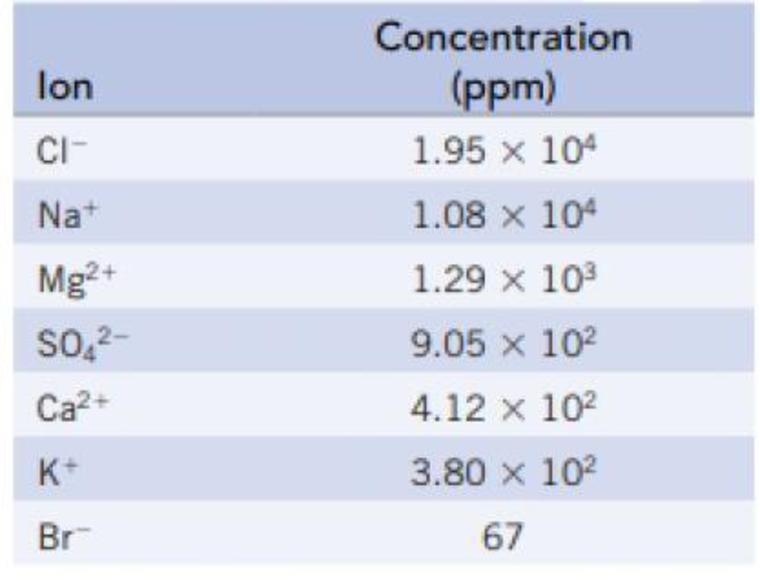# The following table lists the concentrations of the principal ions in seawater: (a) Calculate the freezing point of seawater. (b) Calculate the osmotic pressure of seawater at 25 °C. What is the minimum pressure needed to purify seawater by reverse osmosis?### Chemistry & Chemical Reactivity

9th Edition
John C. Kotz + 3 others
Publisher: Cengage Learning
ISBN: 9781133949640

#### Solutions

Chapter
Section### Chemistry & Chemical Reactivity

9th Edition
John C. Kotz + 3 others
Publisher: Cengage Learning
ISBN: 9781133949640
Chapter 13, Problem 79GQ
Textbook Problem
5 views

## The following table lists the concentrations of the principal ions in seawater:(a) Calculate the freezing point of seawater. (b) Calculate the osmotic pressure of seawater at 25 °C. What is the minimum pressure needed to purify seawater by reverse osmosis?

(a)

Interpretation Introduction

Interpretation: The freezing point of seawater has to be determined.

Concept introduction:

Colligative properties: Properties of solutions which having influence on the concentration of the solute in it. Colligative properties are,

• Decrease in the vapor pressure
• Increase in the boiling point
• Decline in the freezing point
• Osmotic pressure

Freezing point depression: The freezing point of the solution varies with the solute concentration.

Freezing point depression = ΔTfp= Kfp. msolute,where,Kfp=molal freezing point depression constant,msolute= molality of solute.

The number of moles of any substance can be determined using the equation

Numberofmole=GivenmassofthesubstanceMolarmass

### Explanation of Solution

Given,

Molal freezing point depression constant of water is 1.860C/m

The value 1ppm means, it contains 1g of solute in 106g of solution.

Hence, the concentration given in ppm can be taken as the mass of each of the ions on 106g of water.

The number of moles of any substance can be determined using the equation

Numberofmole=GivenmassofthesubstanceMolarmass

NumberofmolesofCl=1.95×104g35.45g/mol=550.0mol

Number of moles of Cl is 550.0mol

NumberofmolesofNa+=1.08×104g22.99g/mol=469.8mol

Number of moles of Na+ is 469.8mol

NumberofmolesofMg+=1.29×103g24.31g/mol=53.06mol

Number of moles of Mg+ is 53.06mol

NumberofmolesofSO43=9.05×102g96.06g/mol=9.421mol

Number of moles of SO43 is 9

(b)

Interpretation Introduction

Interpretation: The osmotic pressure of seawater at 250C has to be determined. Minimum pressure needed to purify the seawater using reverse osmosis method has to be calculated.

Concept introduction:

Colligative properties: Properties of solutions which having influence on the concentration of the solute in it. Colligative properties are,

• Decrease in the vapor pressure
• Increase in the boiling point
• Decline in the freezing point
• Osmotic pressure

Osmotic pressure: The pressure created by the column of solution for the system at equilibrium is a measure of the osmotic pressure and is calculated by using the equation,

π=cRT

where,

c is the molar concentration

The number of moles of any substance can be determined using the equation

Numberofmole=GivenmassofthesubstanceMolarmass

### Still sussing out bartleby?

Check out a sample textbook solution.

See a sample solution

#### The Solution to Your Study Problems

Bartleby provides explanations to thousands of textbook problems written by our experts, many with advanced degrees!

Get Started

Find more solutions based on key concepts
What are cell-cycle checkpoints?

Biology (MindTap Course List)

Most of the time, the buyer is wasting his or her money when buying an ergogenic aid to improve performance. St...

Nutrition: Concepts and Controversies - Standalone book (MindTap Course List)

How do eating disorders affect health?

Understanding Nutrition (MindTap Course List)

What are derived units?

An Introduction to Physical Science

Define the following terms: a. chromosome b. chromatin

Human Heredity: Principles and Issues (MindTap Course List)

What does the designation Pi denote?

Organic And Biological Chemistry

In many kitchens, a microwave oven is used to cook food. The frequency of the microwaves is on the order of 101...

Physics for Scientists and Engineers, Technology Update (No access codes included)

How would Eratostheness estimate have been different if the sun were actually closer to Earth?

Oceanography: An Invitation To Marine Science, Loose-leaf Versin